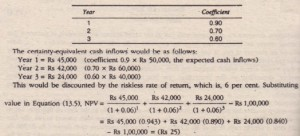Select Page

Accept-Reject Rule

The decision-criterion here can either be the NPV method or the IRR method. Using the NPV method, the proposal would be accepted if the NPV of the certainty-equivalent cash flow is positive, otherwise it would be rejected. If the IRR method is employed, the internal rate of return (r), that equates the present value of certainty-equivalent cash inflows with the present value of the cash outflows, would be compared with the risk-free discount rate. As is the practice with this method, if r exceeds the risk-free rate, the investment project would he accepted. If not, it would be rejected.

The mathematical formulation to give the NPV would be:where a, = certainty-equivalent coefficient for year t

i = riskless interest rate

CO = Cash outflow

We illustrate below the certainty-equivalent approach to adjust risk to capital budgeting analysis on the basis of Example.

Let us further assume that the certainty-equivalent coefficients for future cash inflows in different years are:Since the NPV is negative, the project should be rejected. This decision is in conflict with the decision using the risk-adjusted discount rate where K = 20 per cent. Thus, both these method may not yield identical results.